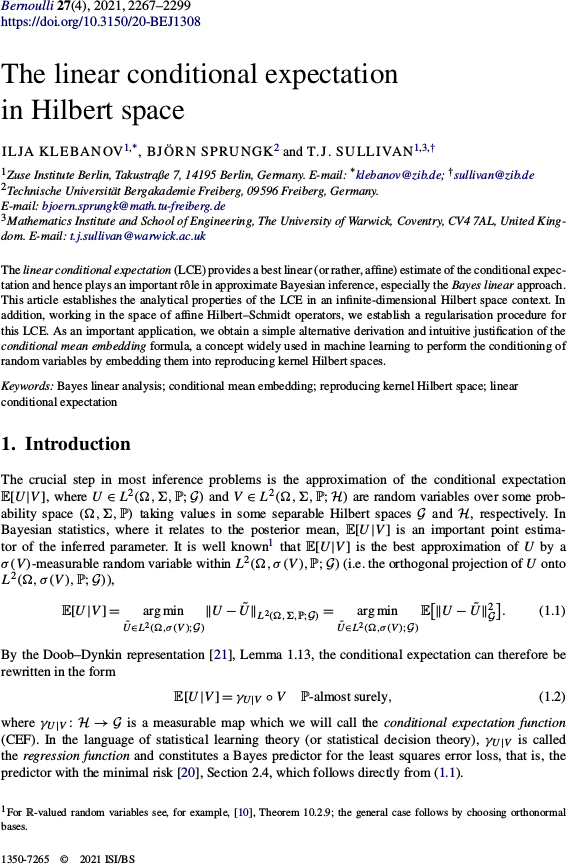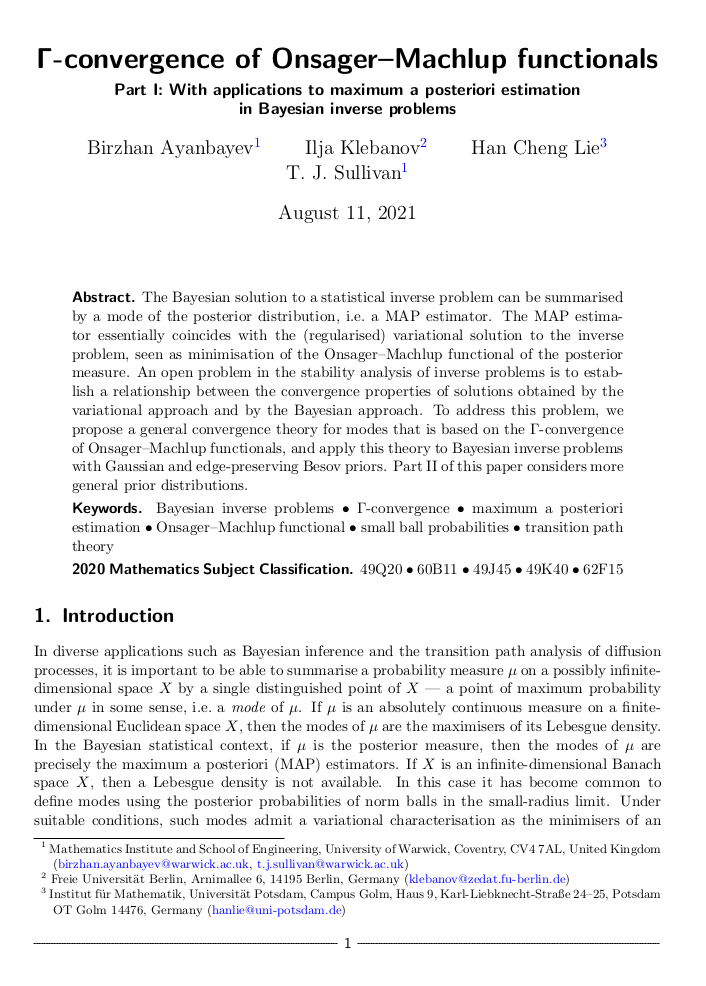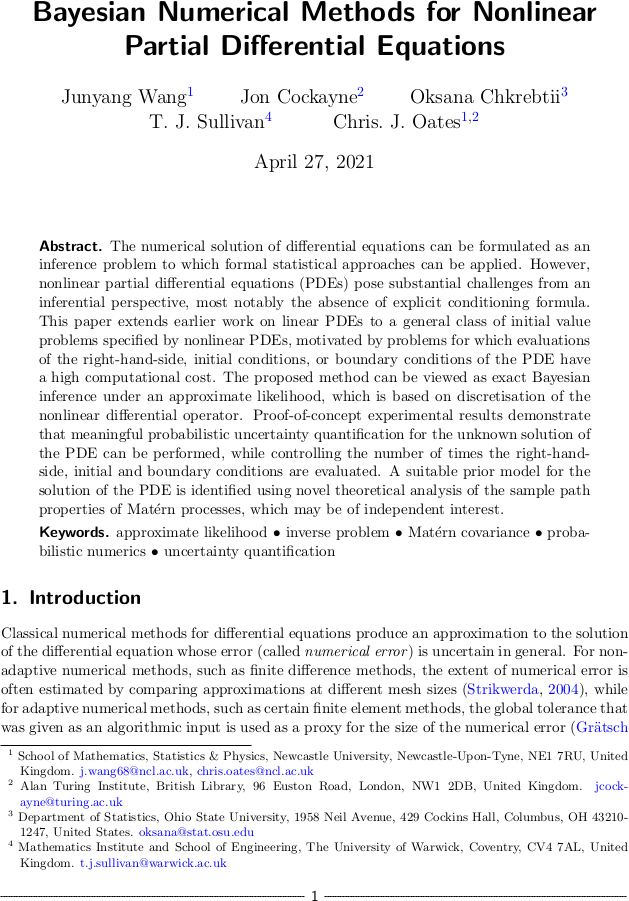# Tim Sullivan

### Welcome!

I am Assistant Professor in Predictive Modelling in the Mathematics Institute and School of Engineering at the University of Warwick. I am also a Turing Fellow at the Alan Turing Institute. I have wide interests in uncertainty quantification the broad sense, understood as the meeting point of numerical analysis, applied probability and statistics, and scientific computation. On this site you will find information about how to contact me, my research, publications, and teaching activities.### Linear conditional expectation in Hilbert space in Bernoulli

The article “The linear conditional expectation in Hilbert space” by Ilja Klebanov, Björn Sprungk, and myself has just appeared in its final form in the journal Bernoulli. In this paper, we study the best approximation $$\mathbb{E}^{\mathrm{A}}[U|V]$$ of the conditional expectation $$\mathbb{E}[U|V]$$ of an $$\mathcal{G}$$-valued random variable $$U$$ conditional upon a $$\mathcal{H}$$-valued random variable $$V$$, where “best” means $$L^{2}$$-optimality within the class $$\mathrm{A}(\mathcal{H}; \mathcal{G})$$ of affine functions of the conditioning variable $$V$$. This approximation is a powerful one and lies at the heart of the Bayes linear approach to statistical inference, but its analytical properties, especially for $$U$$ and $$V$$ taking values in infinite-dimensional spaces $$\mathcal{G}$$ and $$\mathcal{H}$$, are only partially understood — which this article aims to rectify.

I. Klebanov, B. Sprungk, and T. J. Sullivan. “The linear conditional expectation in Hilbert space.” Bernoulli 27(4):2267–2299, 2021. doi:10.3150/20-BEJ1308

Abstract. The linear conditional expectation (LCE) provides a best linear (or rather, affine) estimate of the conditional expectation and hence plays an important rôle in approximate Bayesian inference, especially the Bayes linear approach. This article establishes the analytical properties of the LCE in an infinite-dimensional Hilbert space context. In addition, working in the space of affine Hilbert–Schmidt operators, we establish a regularisation procedure for this LCE. As an important application, we obtain a simple alternative derivation and intuitive justification of the conditional mean embedding formula, a concept widely used in machine learning to perform the conditioning of random variables by embedding them into reproducing kernel Hilbert spaces.

Published on Wednesday 25 August 2021 at 08:00 UTC #publication #tru2 #bayesian #rkhs #mean-embedding #klebanov #sprungk### Γ-convergence of Onsager-Machlup functionals

Birzhan Ayanbayev, Ilja Klebanov, Han Cheng Lie, and I have just uploaded preprints of our work “Γ-convergence of Onsager–Machlup functionals” to the arXiv; this work consists of two parts, “Part I: With applications to maximum a posteriori estimation in Bayesian inverse problems” and “Part II: Infinite product measures on Banach spaces”.

The purpose of this work is to address a long-standing issue in the Bayesian approach to inverse problems, namely the joint stability of a Bayesian posterior and its modes (MAP estimators) when the prior, likelihood, and data are perturbed or approximated. We show that the correct way to approach this problem is to interpret MAP estimators as global weak modes in the sense of Helin and Burger (2015), which can be identified as the global minimisers of the Onsager–Machlup functional of the posterior distribution, and hence to provide a convergence theory for MAP estimators in terms of Γ-convergence of these Onsager–Machlup functionals. It turns out that posterior Γ-convergence can be assessed in a relatively straightforward manner in terms of prior Γ-convergence and continuous convergence of potentials (negative log-likelihoods). Over the two parts of the paper, we carry out this programme both in generality and for specific priors that are commonly used in Bayesian inverse problems, namely Gaussian and Besov priors (Lassas et al., 2009; Dashti et al., 2012).

Abstract (Part I). The Bayesian solution to a statistical inverse problem can be summarised by a mode of the posterior distribution, i.e. a MAP estimator. The MAP estimator essentially coincides with the (regularised) variational solution to the inverse problem, seen as minimisation of the Onsager–Machlup functional of the posterior measure. An open problem in the stability analysis of inverse problems is to establish a relationship between the convergence properties of solutions obtained by the variational approach and by the Bayesian approach. To address this problem, we propose a general convergence theory for modes that is based on the Γ-convergence of Onsager–Machlup functionals, and apply this theory to Bayesian inverse problems with Gaussian and edge-preserving Besov priors. Part II of this paper considers more general prior distributions.

Abstract (Part II). We derive Onsager–Machlup functionals for countable product measures on weighted $$\ell^{p}$$ subspaces of the sequence space $$\mathbb{R}^\mathbb{N}$$. Each measure in the product is a shifted and scaled copy of a reference probability measure on $$\mathbb{R}$$ that admits a sufficiently regular Lebesgue density. We study the equicoercivity and Γ-convergence of sequences of Onsager–Machlup functionals associated to convergent sequences of measures within this class. We use these results to establish analogous results for probability measures on separable Banach or Hilbert spaces, including Gaussian, Cauchy, and Besov measures with summability parameter $$1 \leq p \leq 2$$. Together with Part I of this paper, this provides a basis for analysis of the convergence of maximum a posteriori estimators in Bayesian inverse problems and most likely paths in transition path theory.

Published on Wednesday 11 August 2021 at 12:00 UTC #preprint #inverse-problems #modes #map-estimators #ayanbayev #klebanov #lie### Bayesian numerical methods for nonlinear PDEs

Junyang Wang, Jon Cockayne, Oksana Chkrebtii, Chris Oates, and I have just uploaded a preprint of our recent work “Bayesian numerical methods for nonlinear partial differential equations” to the arXiv. This paper continues our study of (approximate) Bayesian probabilistic numerical methods (Cockayne et al., 2019), in this case for the challenging setting of nonlinear PDEs, with the goal of realising a posterior distribution over the solution of the PDE that carries a meaningful expression of uncertainty about the solution's true value given the discretisation error that has been incurred.

Abstract. The numerical solution of differential equations can be formulated as an inference problem to which formal statistical approaches can be applied. However, nonlinear partial differential equations (PDEs) pose substantial challenges from an inferential perspective, most notably the absence of explicit conditioning formula. This paper extends earlier work on linear PDEs to a general class of initial value problems specified by nonlinear PDEs, motivated by problems for which evaluations of the right-hand-side, initial conditions, or boundary conditions of the PDE have a high computational cost. The proposed method can be viewed as exact Bayesian inference under an approximate likelihood, which is based on discretisation of the nonlinear differential operator. Proof-of-concept experimental results demonstrate that meaningful probabilistic uncertainty quantification for the unknown solution of the PDE can be performed, while controlling the number of times the right-hand-side, initial and boundary conditions are evaluated. A suitable prior model for the solution of the PDE is identified using novel theoretical analysis of the sample path properties of Matérn processes, which may be of independent interest.

Published on Tuesday 27 April 2021 at 10:00 UTC #preprint #prob-num #wang #cockayne #chkrebtii #oates### Computing with dense kernel matrices at near-linear cost in MMS

The paper “Compression, inversion, and approximate PCA of dense kernel matrices at near-linear computational complexity” by Florian Schäfer, Houman Owhadi, and myself has just appeared in print in Multiscale Modeling and Simulation. This paper shows how a surprisingly simple algorithm — the zero fill-in incomplete Cholesky factorisation — with respect to a cleverly-chosen sparsity pattern allows for near-linear complexity compression, inversion, and approximate PCA of square matrices of the form

$$\Theta = \begin{bmatrix} G(x_{1}, x_{1}) & \cdots & G(x_{1}, x_{N}) \\ \vdots & \ddots & \vdots \\ G(x_{N}, x_{1}) & \cdots & G(x_{N}, x_{N}) \end{bmatrix} \in \mathbb{R}^{N \times N} ,$$

where $$\{ x_{1}, \dots, x_{N} \} \subset \mathbb{R}^{d}$$ is a data set and $$G \colon \mathbb{R}^{d} \times \mathbb{R}^{d} \to \mathbb{R}$$ is a covariance kernel function. Such matrices play a key role in, for example, Gaussian process regression and RKHS-based machine learning techniques.

F. Schäfer, T. J. Sullivan, and H. Owhadi. “Compression, inversion, and approximate PCA of dense kernel matrices at near-linear computational complexity.” Multiscale Modeling & Simulation: A SIAM Interdisciplinary Journal 19(2):688–730, 2021. doi:10.1137/19M129526X

Abstract. Dense kernel matrices $$\Theta \in \mathbb{R}^{N \times N}$$ obtained from point evaluations of a covariance function $$G$$ at locations $$\{ x_{i} \}_{1 \leq i \leq N}$$ arise in statistics, machine learning, and numerical analysis. For covariance functions that are Green's functions of elliptic boundary value problems and homogeneously-distributed sampling points, we show how to identify a subset $$S \subset \{ 1 , \dots , N \}^2$$, with $$\# S = O ( N \log (N) \log^{d} ( N /\varepsilon ) )$$, such that the zero fill-in incomplete Cholesky factorisation of the sparse matrix $$\Theta_{ij} 1_{( i, j ) \in S}$$ is an $$\varepsilon$$-approximation of $$\Theta$$. This factorisation can provably be obtained in complexity $$O ( N \log( N ) \log^{d}( N /\varepsilon) )$$ in space and $$O ( N \log^{2}( N ) \log^{2d}( N /\varepsilon) )$$ in time; we further present numerical evidence that $$d$$ can be taken to be the intrinsic dimension of the data set rather than that of the ambient space. The algorithm only needs to know the spatial configuration of the $$x_{i}$$ and does not require an analytic representation of $$G$$. Furthermore, this factorization straightforwardly provides an approximate sparse PCA with optimal rate of convergence in the operator norm. Hence, by using only subsampling and the incomplete Cholesky factorization, we obtain, at nearly linear complexity, the compression, inversion and approximate PCA of a large class of covariance matrices. By inverting the order of the Cholesky factorization we also obtain a solver for elliptic PDE with complexity $$O ( N \log^{d}( N /\varepsilon) )$$ in space and $$O ( N \log^{2d}( N /\varepsilon) )$$ in time.

Published on Thursday 15 April 2021 at 12:00 UTC #publication #prob-num #schaefer #owhadi### Randomised integration for deterministic operator differential equations

Han Cheng Lie, Martin Stahn, and I have just uploaded a preprint of our recent work “Randomised one-step time integration methods for deterministic operator differential equations” to the arXiv. In this paper, we extend the analysis of Conrad et al. (2016) and Lie et al. (2019) to the case of evolutionary systems in Banach spaces or even Gel′fand triples, this being the right setting for many evolutionary partial differential equations.

Abstract. Uncertainty quantification plays an important role in applications that involve simulating ensembles of trajectories of dynamical systems. Conrad et al. (Stat. Comput., 2017) proposed randomisation of deterministic time integration methods as a strategy for quantifying uncertainty due to time discretisation. We consider this strategy for systems that are described by deterministic, possibly non-autonomous operator differential equations defined on a Banach space or a Gel′fand triple. We prove pathwise and expected error bounds on the random trajectories, given an assumption on the local truncation error of the underlying deterministic time integration and an assumption that the absolute moments of the random variables decay with the time step. Our analysis shows that the error analysis for differential equations in finite-dimensional Euclidean space carries over to infinite-dimensional settings.

Published on Wednesday 31 March 2021 at 09:00 UTC #preprint #prob-num #lie #stahn